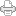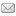Forming Atoms

Posted on Monday, September 29, 2008 at 06:45AM

In the last entry, we announced the breakthrough, following Montgomery and Jeffrey (M&J), that allows us to form atoms from nothing but motion. In our case, time and space speed-displacements (SUDRs & TUDRs) join to form biforms called S|T units. These S|T units combine to form triforms, which act as preons to form two basic types of combinations called bosons and fermions. Bosons are photons, while fermions are quarks, electrons and neutrinos, each with their “antiparticles.”

The way M&J’s model works, three quarks constitute a triform, depending on the mix of quarks. With two up and one down quark, a proton can be fitted to a neutron, with two down and one up quark, in such a way that the up and down quarks constitute bonded pairs at the three apexes of two triangles in two planes. Combining a proton & electron, H, with a neutron, forms deuterium, 2H, and adding two deuterium atoms together forms a hexagonal lattice of helium, 4H, or the alpha particle, which then becomes the basic building block of their table of nuclides.

However, following nuclear reactions, tritium, 3H and 3He are formed first, as intermediate stages, by adding another neutron to 2H, which decays in about 12 years to 3He, but just how this works in the model is not so clear. Moreover, in the M&J model, the bonds between the constituent quarks are formed from electrical and magnetic forces, which are not used per se in an RST model, since force is defined as a property of motion.

These considerations have forced us to take another path in the development of the periodic table of elements that modifies the way the constituent quarks of the protons and neutrons are paired to form higher combinations, compared to the M&J model. Instead of forming a Dagwood sandwich from a deuterium atom, by adding a neutron on the other side of the proton, sandwiching it in between the two neutrons, in tritium, which decays into a proton|neutron|proton sandwich of helium three, we, again, form a triangle of triangles, following the quark pattern of triangles within a greater triangle.

Thus, in the LRC’s RSt model, quark triangles form proton and neutron triangles, and, now, these nuclei triangles form atomic triangles, as tritium and three helium. Of course, we have to remember that these triangles are only 2D diagrams, or schematics of the physical triangles of 3D merged spheres, but otherwise, it works out well.

Recall, that another difference in our model is that we include electrons with the quarks of protons, so we are dealing with atoms, not atomic nuclei, which don’t exist in our model. With only motion to work with, we have only two opposite, or dual, quantities to form the bonds between quarks, time motion and space motion ( time-displaced units = SUDRs and space-displaced units = TUDRs).

When deuterium forms, the total space and time units of motion balance out, which can be expressed as shown in figure 1 below:Figure 1. Quarks, Nuclei and Deuterium

As shown in figure 1 above, the quarks bond perfectly as the proton|neutron mirror one another, with the electron sandwiched in between them, and the number of positive units equals the number of negative units. However, where do we go from here? As depicted in the bottom right panel of the figure, deuterium has no way to bond to deuterium. The polarity of the motion combinations match, so we seem to be stuck.

In the M&J model, the alpha particle, helium four, is formed from this latter combination of deuterium, by postulating that electrical and magnetic forces exist between the deuterium nuclei sufficient to bond them together (no mention is made of the electroweak force, only the electromagnetic force, as far as I can determine). Subsequently, once the deuterium atom is formed in this manner, another nucleon is added to the appropriate mirror nucleon to begin the formation of another alpha component (with tritium and helium three, as intermediaries, although tritium is not shown on the website)

Nevertheless, it’s clear from figure 1 that we cannot follow that procedure in our model, using motion combinations. Fortunately, though, if we re-arrange the nuclei triangles, so that, instead of mirroring them, as two layered planes, as shown in figure 1, we connect the nodes in a planar array of triangles, as shown in figure 2 below, we not only can form tritium and helium three, but helium four as well, and, indeed, every other isotope combination in the table of nuclides.Figure 2. Planar Array of Nuclides Tritium and Helium Three

Since down quarks are composed of two green (balanced) S|T units and one red S|T unit (i.e. red = more SUDRs than TUDRs,) the unbalance is one red unit, or -1, while in the up quarks, there are two blue units with one green unit, for an unbalance of 2 blue units, or +2. With the three red units, -3, of the electron, the polarities balance out for each isotope, no matter how many protons and neutrons an atom is composed of. Therefore, we can simplify the diagrams by using colored triangles, blue for protons, and green for neutrons. Assuming that each node is an U-D quark bond, we can obviously diagram any atom in a planar array of triangles arranged on the sides of a polygon, with the requisite number of sides corresponding to the total number of protons and neutrons making up the isotope, as illustrated in Figure 3 below.Figure 3. Schematic Diagram of LRC’s Model of Periodic Elements

Of course, there’s much more to the model than just a data schema, such as physical parameters that can account for physical properties, especially periodicity, mass and absoption/emission spectra, but then this is great progress.View Printer Friendly VersionEmail Article to Friend